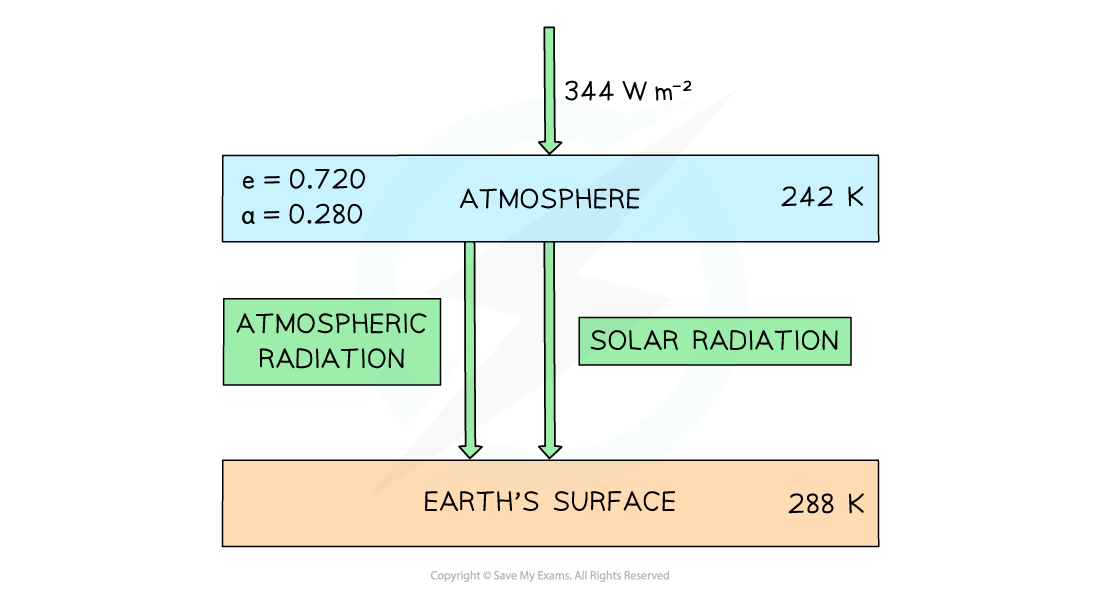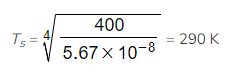# IB DP Physics: HL复习笔记8.2.5 Earth's Surface–Atmosphere System

### Earth's Surface–Atmosphere System

• It is useful to consider Earth’s energy balance in terms of how much incoming energy from the Sun is used and how much is returned to space
• If incoming and outgoing energy are in balance, the Earth’s temperature will remain constant
• This can be used to create models which can help climate scientists predict temperature fluctuations based on current and increased concentrations of greenhouse gases
• At it’s simplest, the model involves a one-layer atmosphere above the Earth’s surface

#### Worked Example

The diagram below shows a simple energy balance climate model in which the atmosphere and the Earth’s surface are treated as two bodies.At current atmospheric greenhouse gas concentrations, the temperature of Earth’s atmosphere is set to increase by 6 K.

Data for this model:

• Current mean temperature of the Earth’s atmosphere = 242 K
• Current mean temperature of the Earth’s surface = 288 K
• Solar power per unit area at top of the atmosphere = 344 W m–2
• Emissivity of the atmosphere, e = 0.720
• Albedo of the atmosphere, a = 0.280

Use this data to estimate the increase in temperature of the Earth’s surface.

Step 1: List the known quantities

• Solar power above atmosphere, Pa = 344 W m–2
• Emissivity of the atmosphere, e = 0.720
• Emissivity of the surface, e = 1
• New temperature of Earth’s atmosphere, Ta = 242 + 6 = 248 K
• Stefan-Boltzmann constant, σ = 5.67 × 10–8 W m–2 K–4
• Power absorbed at the Earth’s surface = Ps
• New temperature of Earth’s surface = Ts

Step 2: Calculate the solar power absorbed at the Earth’s surface

• This can be calculated using the emissivity and the solar power above the atmosphere

Ps = e × Pa

Ps = 0.720 × 344 = 247.68 = 248 W m–2

Step 3: Write the equation for the power emitted by a body

P = eσT4

Step 4: Calculate the new power radiated by the atmosphere

P = 0.720 × (5.67 × 10–8) × 2484 = 154.43 = 154 W m–2

Step 5: Calculate the new power absorbed by the Earth’s surface

• The power absorbed by the Earth’s surface is a sum of the solar radiation that reaches the surface plus the power radiated by the atmosphere

New power, Ps = 248 + 154 = 402 W m–2

Step 6: Calculate the new temperature of the Earth’s surface

• The Earth’s surface can be assumed to be a black body, hence e = 1

Ps = σTs4

400 = (5.67 × 10–8) × Ts4Step 7: Determine the increase in temperature

ΔT = 290 – 288 = 2 K

#### Exam Tip

In simplified climate models, you can generally assume the Earth’s surface and the atmosphere:

• Act as black bodies - this means the emissivity of the surface will be equal to 1!
• Remain at a constant temperature